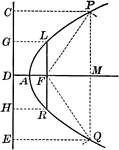### Parabola With Focus and Directrix

Illustration of a parabola - a curve which is the locus of a point that moves in a plane so that its…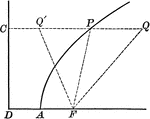### Point on Parabola

Illustration of a parabola showing point P is equidistant to the focus and the directrix.### Point on Parabola

Illustration of a parabola. "If a line PT is drawn from any point P of the curve, bisecting the angle…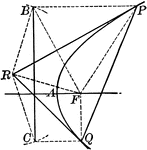### Tangent to a Parabola

Illustration of a tangent line drawn from an external point to a parabola.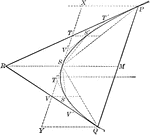### Tangents and Chords of a Parabola

Illustration showing that the area of a parabolic segment made by a chord is two thirds the area of…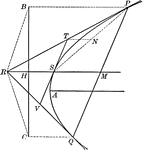### Tangents to a Parabola

Illustration showing that if two tangents RP and RQ are drawn from a point R to a parabola, the line…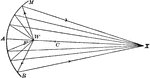### Reflection of Sound Using a Reflector

"Hold a lamp reflector or other large concave mirror directly facing the sun, so as to bring the rays…